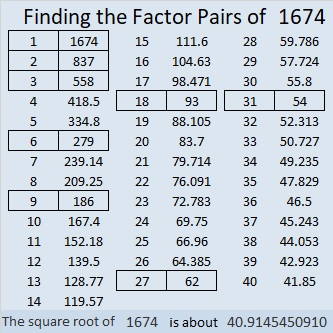# 1674 and Level 2

Contents

### Today’s Puzzle:

Make a multiplication table out of this puzzle. Can you see how to do it? The factors won’t be in the usual order, but I’m sure you can figure it out!### Factors of 1674:

• 1674 is a composite number.
• Prime factorization: 1674 = 2 × 3 × 3 × 3 × 31, which can be written 1674 = 2 × 3³ × 31.
• 1674 has at least one exponent greater than 1 in its prime factorization so √1674 can be simplified. Taking the factor pair from the factor pair table below with the largest square number factor, we get √1674 = (√9)(√186) = 3√186.
• The exponents in the prime factorization are 1,3 and 1. Adding one to each exponent and multiplying we get (1 + 1)(3 + 1)(1 + 1) = 2 × 4 × 2 = 16. Therefore 1674 has exactly 16 factors.
• The factors of 1674 are outlined with their factor pair partners in the graphic below.### More about the number 1674:

From OEIS.org we learn that
1/1 + 1/2 + 1/3 + 1/4 + . . . + 1/1672 + 1/1673 ≈ 7.999888, but if you add the next tiny fraction, 1/1674, the sum will be a tiny bit more than 8 or approximately 8.000486.

That’s adding a whole lot of unit fractions just to get a sum over 8.

This site uses Akismet to reduce spam. Learn how your comment data is processed.